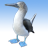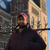# QlikView App Dev

Discussion Board for collaboration related to QlikView App Development.

cancel
Showing results for
Did you mean:Creator

## Set Analysis Within Set Analysis (Qlik Sense)

Hey everyone,

I am working with a set of banking records. I need to be able to count the closed accounts where their close date is within the last month and does NOT have a closing balance of zero. I am able to get the count of the closed within the last month with the following set analysis:

Count({ <Deposits_close_dt={">\$(= Date(MonthEnd(AddMonths(GetFieldSelections(([Production Date])),-1)), 'YYYY-MM-DD')) <= \$(= Date(([Production Date])))"}> }Distinct acct_nbr)

So I am wondering, do any of you know how to then filter these account to show the count where their closing balances are not zero. I tried an if statement with the above set analysis as the then section, but got no result. Would I be able to do a set analysis within a set analysis? I will try to include some sample data. Any help is appreciated!

Thank you,

Liam Grover

1 Solution

Accepted SolutionsChampion

Hi,

try this:

Count({ <Deposits_close_dt={">\$(= Date(MonthEnd(AddMonths(GetFieldSelections(([Production Date])),-1)), 'YYYY-MM-DD')) <= \$(= Date(([Production Date])))"},acct_nbr={"=sum([closing balances])>0"}> }Distinct acct_nbr)

PS: verify your closing balances field name

4 RepliesChampion

Hi,

try this:

Count({ <Deposits_close_dt={">\$(= Date(MonthEnd(AddMonths(GetFieldSelections(([Production Date])),-1)), 'YYYY-MM-DD')) <= \$(= Date(([Production Date])))"},acct_nbr={"=sum([closing balances])>0"}> }Distinct acct_nbr)

PS: verify your closing balances field nameMVP

May be this

Count({<Deposits_close_dt = {">\$(=Date(MonthEnd(AddMonths(GetFieldSelections(([Production Date])),-1)), 'YYYY-MM-DD'))<=\$(=Date(([Production Date])))"}, [Closing Balance] = {'>0'}>} Distinct acct_nbr)Creator
Author

Perfect, Thanks for the fast response!Champion

You're welcome